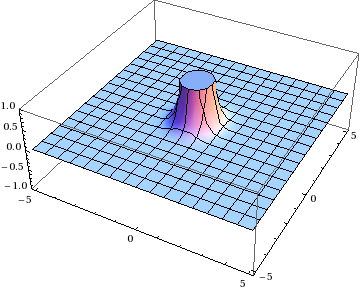1. 2012
Nov
20

## A simple regularization exampleEarlier this month I promised to show you a simple example of regularization. Well, here it is. This is a particular combination of integrals I’ve been working with a lot lately:

$$\iint_{\mathbb{R}^2} \uddc\mathbf{x}\frac{e^{-x^2}}{x^2}e^{-i\mathbf{k}\cdot\mathbf{x}} - \iint_{\mathbb{R}^2} \frac{\uddc\mathbf{y}}{y^2}e^{-i\xi\mathbf{k}\cdot\mathbf{y}}$$

A quick look at the formulas shows you that both integrands have singularities at $$\mathbf{x} = 0$$ and $$\mathbf{y} = 0$$.

OK, well, that’s why we have two integrands. We can change variables from $$\xi\mathbf{y}\to\mathbf{x}$$, subtract them, and the singularities will cancel out, right? You can do this integral by hand in polar coordinates, or just pop it into Mathematica:

$$\iint_{\mathbb{R}^2} \uddc\mathbf{x}\frac{e^{-x^2} - 1}{x^2}e^{-i\mathbf{k}\cdot\mathbf{x}} = -\pi\Gamma\biggl(0, \frac{k^2}{4}\biggr)$$

Just one problem: that’s not the right answer! You can tell because the value of this integral had better depend on $$\xi$$, but this expression doesn’t. This isn’t anything so mundane …

2. 2012
Nov
04

## Not really a simple regularization analogy

Last year I posted about an infinite sum involving the mean of the harmonic numbers,

$$\lim_{n\to\infty}\frac{1}{n}\sum_{k=1}^{n}\frac{1}{k} = \lim_{n\to\infty}\frac{\ln n}{n}$$

The method I used to evaluate this was to approximate the sum by an integral. This is a technique that is used in various places in physics, such as in the computation of the Fermi surface in metals.

In this particular case, we only cared about the large-$$n$$ limiting behavior of the sum, namely that it grows sublinearly for large $$n$$. But suppose you wanted to know whether there was, say, a constant term as well. Here’s one way to figure that out. Instead of converting the entire sum to an integral, you choose some cutoff value $$a$$, and keep the first $$a$$ terms in the sum explicitly.

$$\sum_{k=1}^{n}\frac{1}{k} \approx \sum_{k=1}^{a}\frac{1}{k} + \sum_{k=a+1}^{n}\frac{1}{k} = \sum_{k=1}^{a}\frac{1}{k} + \ln\frac{n}{a+1}$$

Now you have a value, $$a$$, that represents a “break” in your sequence, but it’s an arbitrary …